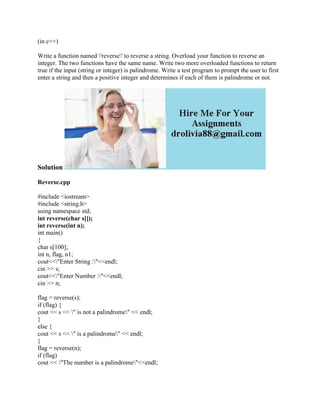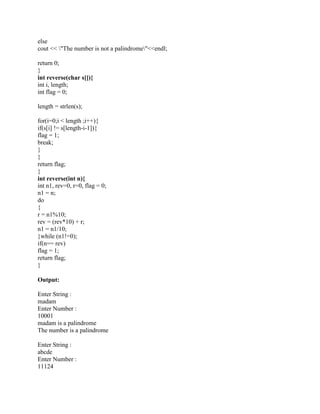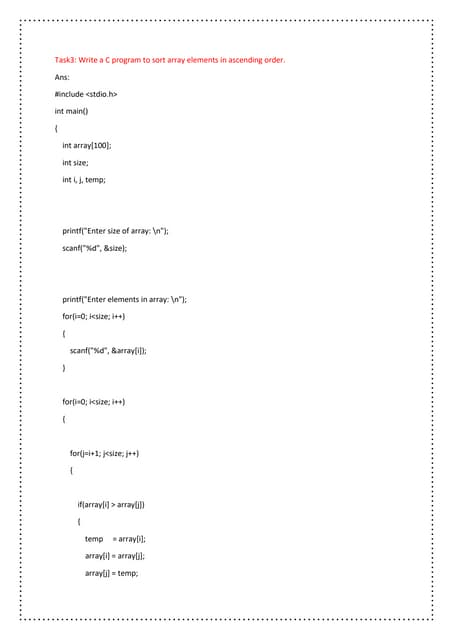Anúncio

# (in c++) Write a function named -'reverse-' to reverse a string- Overl.docx

7 de Feb de 2023Próximos SlideSharesBasic C Programming Lab Practice
Carregando em ... 3
1 de 3
Anúncio

### (in c++) Write a function named -'reverse-' to reverse a string- Overl.docx

1. (in c++) Write a function named 'reverse' to reverse a string. Overload your function to reverse an integer. The two functions have the same name. Write two more overloaded functions to return true if the input (string or integer) is palindrome. Write a test program to prompt the user to first enter a string and then a positive integer and determines if each of them is palindrome or not. Solution Reverse.cpp #include <iostream> #include <string.h> using namespace std; int reverse(char s[]); int reverse(int n); int main() { char s; int n, flag, n1; cout<<"Enter String :"<<endl; cin >> s; cout<<"Enter Number :"<<endl; cin >> n; flag = reverse(s); if (flag) { cout << s << " is not a palindrome" << endl; } else { cout << s << " is a palindrome" << endl; } flag = reverse(n); if (flag) cout << "The number is a palindrome"<<endl;
2. else cout << "The number is not a palindrome"<<endl; return 0; } int reverse(char s[]){ int i, length; int flag = 0; length = strlen(s); for(i=0;i < length ;i++){ if(s[i] != s[length-i-1]){ flag = 1; break; } } return flag; } int reverse(int n){ int n1, rev=0, r=0, flag = 0; n1 = n; do { r = n1%10; rev = (rev*10) + r; n1 = n1/10; }while (n1!=0); if(n== rev) flag = 1; return flag; } Output: Enter String : madam Enter Number : 10001 madam is a palindrome The number is a palindrome Enter String : abcde Enter Number : 11124
3. abcde is not a palindrome The number is not a palindrome
Anúncio